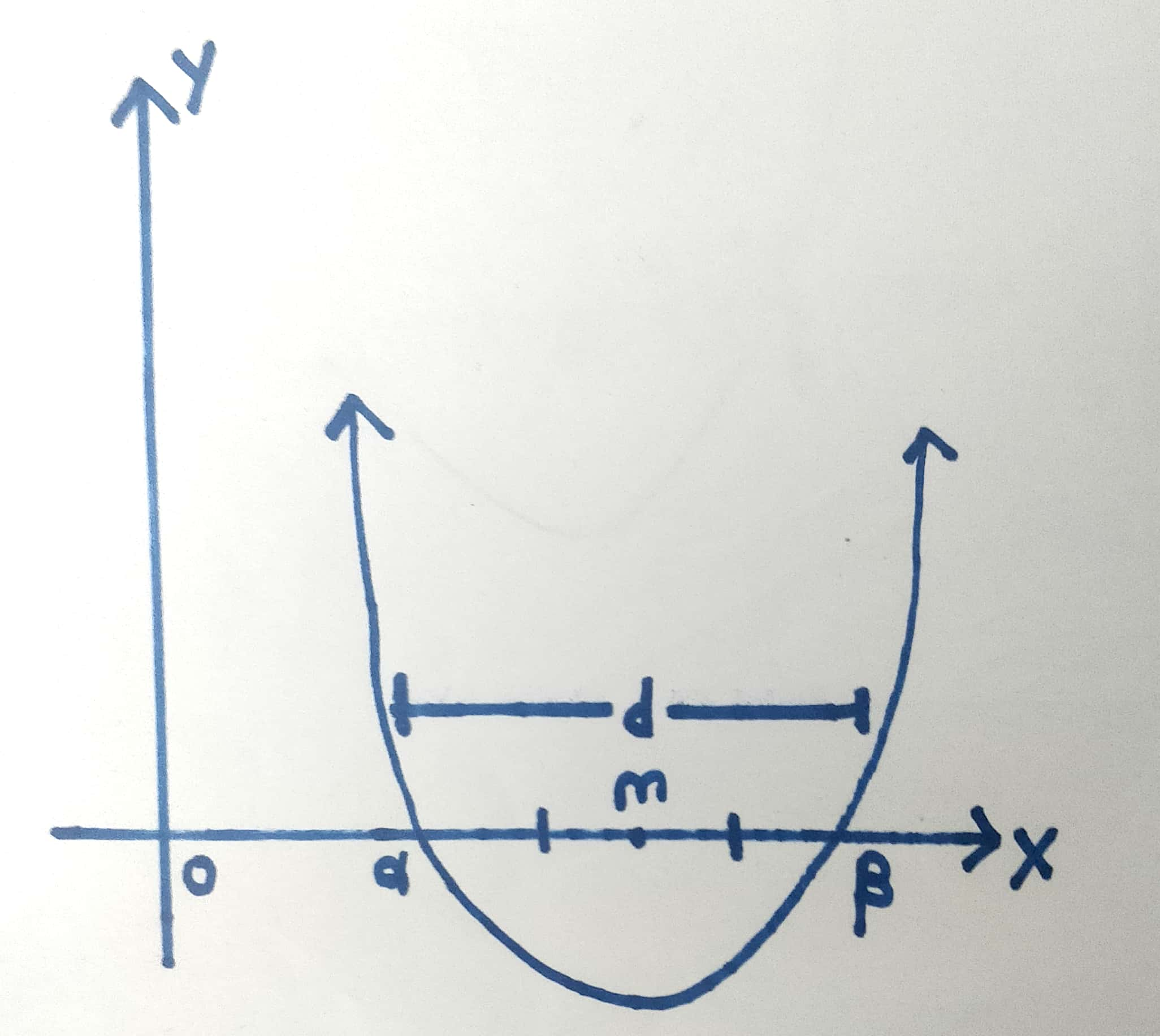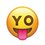# A Better Way to Solve a Quadratic Equation

Hello everyone. Here is an alternate(somewhat simpler) method of solving a quadratic equation. I learnt it from a youtube stream by "3 Blue 1 Brown". The method was originally given by Po Shen Loh.

The Method is Below:

We all know that the equation of the form $ax^{2}+bx+c=0$ is known as a quadratic equation and the formula for its roots is $\frac{-b \pm \sqrt{b^{2}-4ac}}{2a}$.
But there is a shorter formula which is much easier to use while solving problems. You just have to know the three facts below and then after a little manipulation, you are done!

Fact 1: sum of roots is $\frac{-b}{a}$
Fact 2: product of roots is $\frac{c}{a}$
Fact 3: difference of squares i. e. $(m-d)(m+d)=m^{2}-d^{2}$

Let's introduce 2 new variables:
1) $d =$ difference between the 2 roots . i . e $|a-b|$
2) $m =$ mean of the 2 roots . i . e $\frac{a+b}{2}$
In the graph below, notice that $\alpha\beta= (m+d)(m-d) = m^{2}-d^{2}=\frac{c}{a}$ $(\alpha$ and $\beta$ are the 2 roots) because $\alpha = m+d$ and $\beta = m-d$.
So $d^{2}= m - p$ where p is the product of the 2 roots.
Hence, the 2 roots are $m \pm \sqrt{m^{2}-p}$

The formula for the roots of a quadratic equation $m \pm \sqrt{m^{2}-p}$ where p is the product of roots and m is the midpoint of the roots which can be derived from the 3 above facts.

Now, I will do an example of this: Solve the equation $x^{2}+3x+1=0$

We have $m=\frac{-3}{2}$ and $p=\frac{1}{2}$
Hence, by our above formula $x = \frac{-3}{2} \pm \frac{\sqrt{5}}{2}$

The traditional quadratic formula can be derived from this and vice versa. If you have any questions or suggestions, please ask them in the comments. Try more examples to get the hang of it. Hope you liked this note and will start using this formula in the future!

Diagram is belowNote by Nitin Kumar
1 year, 3 months ago

This discussion board is a place to discuss our Daily Challenges and the math and science related to those challenges. Explanations are more than just a solution — they should explain the steps and thinking strategies that you used to obtain the solution. Comments should further the discussion of math and science.

When posting on Brilliant:

• Use the emojis to react to an explanation, whether you're congratulating a job well done , or just really confused .
• Ask specific questions about the challenge or the steps in somebody's explanation. Well-posed questions can add a lot to the discussion, but posting "I don't understand!" doesn't help anyone.
• Try to contribute something new to the discussion, whether it is an extension, generalization or other idea related to the challenge.

MarkdownAppears as
*italics* or _italics_ italics
**bold** or __bold__ bold
- bulleted- list
• bulleted
• list
1. numbered2. list
1. numbered
2. list
Note: you must add a full line of space before and after lists for them to show up correctly
paragraph 1paragraph 2

paragraph 1

paragraph 2

[example link](https://brilliant.org)example link
> This is a quote
This is a quote
    # I indented these lines
# 4 spaces, and now they show
# up as a code block.

print "hello world"
# I indented these lines
# 4 spaces, and now they show
# up as a code block.

print "hello world"
MathAppears as
Remember to wrap math in $$ ... $$ or $ ... $ to ensure proper formatting.
2 \times 3 $2 \times 3$
2^{34} $2^{34}$
a_{i-1} $a_{i-1}$
\frac{2}{3} $\frac{2}{3}$
\sqrt{2} $\sqrt{2}$
\sum_{i=1}^3 $\sum_{i=1}^3$
\sin \theta $\sin \theta$
\boxed{123} $\boxed{123}$

Sort by:

Lockdown Math series is so fun!

- 1 year, 2 months ago

Except for the horrible time. I sleep, wake up and then sleep again as it's at 12. Lol

- 1 year, 2 months ago

I just watch it after it ends. I can't concentrate when I'm sleepy. And my parents won't allow me to wake watch a livestream at midnight.

- 1 year, 2 months ago

Yeah, Grant should try to change the time as many Indians are watching the stream. Even I stopped watching them live as I am tired for the rest of the day.

- 1 year, 2 months ago

Yup it is

- 1 year, 2 months ago

Thanks, @Nitin Kumar. Really helps!

- 1 year, 2 months ago

Also, proof of derivation would be extremely nice! I love this discussion!

- 1 year, 2 months ago

what do you mean by "proof of derivation"?

- 1 year, 2 months ago

Proof of derivation means : how did you derive your simplified quadratic formula from the quadratic formula? Also, still waiting for the graph!

- 1 year, 2 months ago

I put the graph! OK , Ill put the derivation.

- 1 year, 2 months ago

- 1 year, 2 months ago

Nice, @Nitin Kumar. Can you show the graph.

- 1 year, 2 months ago

ok, sure!

- 1 year, 2 months ago

The graph is a general parabola.

- 1 year, 3 months ago